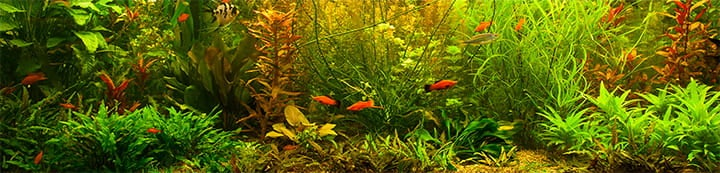1 - 1 of 1 Posts

#### Piotr Sroczyński

·
##### Registered
Joined
·
10 Posts
Discussion Starter
A few days ago, when measured hardness of water - KH and GH, I hit into the idea of a substantial resolution increase, without any additional indicator use.

The original instructions for my test looks like this:
take 5ml of the water and then add a drop of indicator everything stirring. If, after adding a drop the color change the number of drops is equal the hardness of water (in german degrees). In this guide is also written that we can increase the accuracy of the test by doubling tested water (10ml) and the result we obtain by dividing the number of drops by two.

My idea is this:
1. Follow closely by the above instructions to determine water hardness.
2. Treat what you take as an upper limit of hardness and then
add to the test tube by drop the tested water stirring thoroughly. This
time we are waiting for the withdrawal of the colors. Remember the number of
drops given to the transition.
3. Hardness are counting on the basis of the formula:
dH = number_of_indicator_drops * 5ml / (5ml + number_of_added_water / 20)
assuming that 20 drops equals to a 1ml volume and the primary tested water volume is 5ml.
4. Obtained by this measurement resolution is approximately 1% of
number_of_indicator_drops so 0.1dH for 10dH or 0.01dH for 1DH.
It follows to the accuracy of applying 1 drop = 0.05 ml

At the base of this reasoning is the assumption that reaction occuring is reversible in color change.

Piotr

1 - 1 of 1 Posts